# Smash The Code Scoring Question

So I have the silver boss about to drop a green 2/2 block at column 4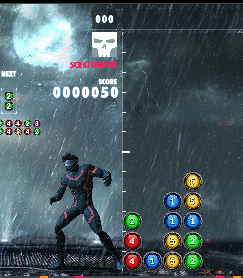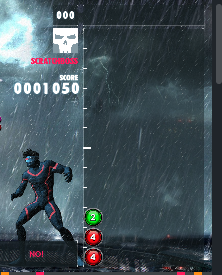He scores 1000 points, how is this calculated?
The blocks collapse 4 greens, then 4 yellows, then 4 blues
From the instructions I get
B = 4, different colours=3 so CB = 4, The Chain is 3 so CP=16, Group bonus=? not 100% on this but if it is all the blocks of which there are 12 this scores GB=8 Total score B10(CB+CP+GB) which is 1120, but he scored 1000 which implies GB should have been 9 blocks, which scores 5
What am I doing wrong?

Next: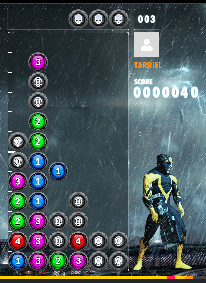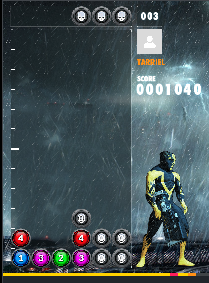I have just dropped the pink 3 and blue 1 and also scored 1000, the cascade I think was 4 blue, along with 3 skulls, 4 green, along with 1 skull, 4 pink, along with 3 skulls. It says that the skulls are like colours except dropping and clearing them, so
B=7, The Chain is 3 so CP=16, different colours=4 so CB = 8, Group bonus=? guessing at 19 which scores GB=8, for a total of 2240, turning the dropped skulls to my advantage but that isn’t the score I got, infact GB can be 0 and I still score 1680, so I need to ignore the white stones and come up with the same as before?
But then…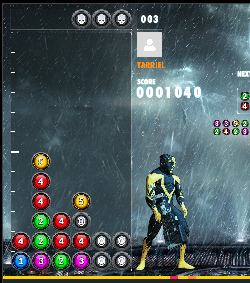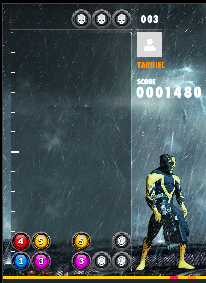scores 440 points, which I get B=8,CP=8(2 steps), CB=4(red,green,white), GB=8 scores 1600,
so ignoring the skulls, and letting GB=0, I still get B=6,CP=8(2 steps),CB=2 (Red,green) which is a score of 600 and I only got 440

Clearly I am doing something wrong. Can someone please explain how the scoring actually works, as I am finally at a place where this is starting to matter. There is a big difference in game state with a score of 440 and 1600

The score of each step is calculated separately.

For example in your first case, the boss do those clear in 3 steps:
s1: 4 green: 4101(minimal CP+CB+GP) pt
s2: 4 yellow: 4108(8 CP) = 320
s3: 4 blue: 41016 (16 CP) = 640
sums to 1000~

1 Like

I did consider this, but I still couldn’t get that to work. So is the group bonus 0 for all three steps?

the second example?
CB=2 for all 3 steps, and GB=?, assume 0 (unless it can go negative?)
S1: 710( 2 CB) = 140
S2: 510(2 CB+ 8 CP)=500
S3: 710(2 CB+ 16 CP)=1260
sums to 1900, rather than 1000

the third:
S1: 810(2 CB) = 160
S2: 410(8 CP) = 320
Sums to 480, rather than 440

so even ignoring the group bonus I am ending up too high. Providing I have done my maths correctly… This is rather confusing. Even ignoring the skulls example 3 still ends up at 430 rather than 440 so I still don’t understand something, and this is without including the group bonus

OK, so if I ignore the skull as a colour, or block, and consider the group bonus for the current step I can get example 3 to work
S1: 610(2 GB) = 120
S2: 410(8 CP) = 320
sums to 440 Is this correct?

I think that if you don’t count the skulls cleared into B and a color, you would get the right answerAnd thank you for helping me figure out a bug in my bot

Yes, this is exactly how this works. Your last calculations are OK.

(In the second example, if you ignore the skulls it behaves just like the first ofc.)
Skulls do not count anywhere - you are just happy they are gone.

Thanks for your help, I think they should change their description a little as it was not really obvious to me. In fact they tell me the skulls are colours just harder to eliminate.

##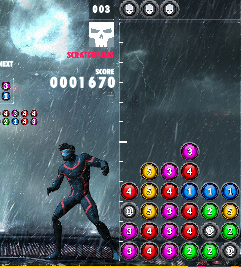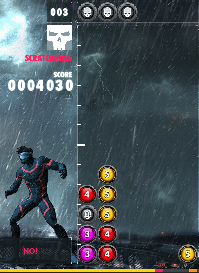s1: 4 blue: 4101(minimal CP+CB+GP) = 40
s2: 4 red : 4108(8 CP) = 320
s3: Group blocks total is 10, which scores 6
6 pink: 610(16 CP+2 CB+6 GB)=1440
4 yellow: 410(16 CP+2 CB+6 GB)=960
for a total of 2760 but we only got a score of 2360

so what am I doing wrong here?
other things I tested… if I assume a group of 6 for the pink, then a group of 10 for the yellow it gives 2520, if I assume 4 for yellow and 10 for pink I get the same, groups of 6 for pink and 4 for yellow I get 2280

step3: you got
10 * 10 * (16 CP + 2CB + 2GB) = 2000

Since you only calculate by this formula once per step.
And your GB is calculated per group and then summed up( so 2 + 0 for those 2 groups )

Thanks, So GB is calculated each step and summed not the blocks summed then calculated, I have a working scorer now (I think).